# 数据挖掘学习05 - 使用R对文本进行hierarchical cluster并验证结果

 1 2 cd = read.table("d:/cluster_data.txt", header = TRUE); attributes(cd);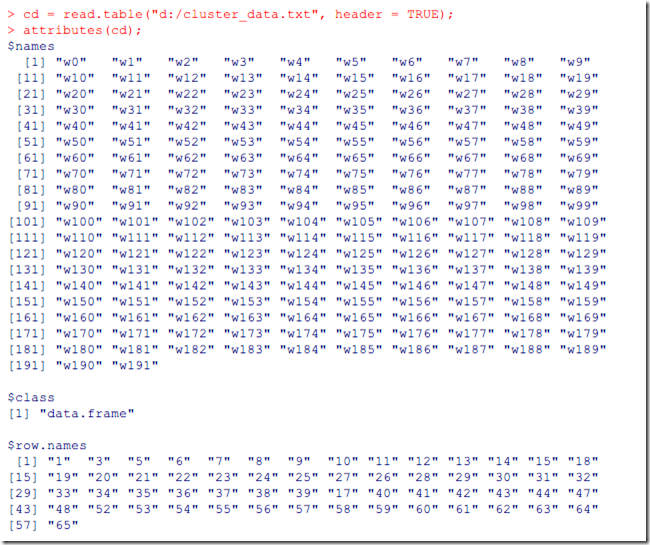cd是一个特征举证，每一行是文档的id和此文档的特征向量，代表一个文本，列代表的是词语，这里用wN代替。

 1 2 cds = dist(cd, method="euclidean") attributes(cds)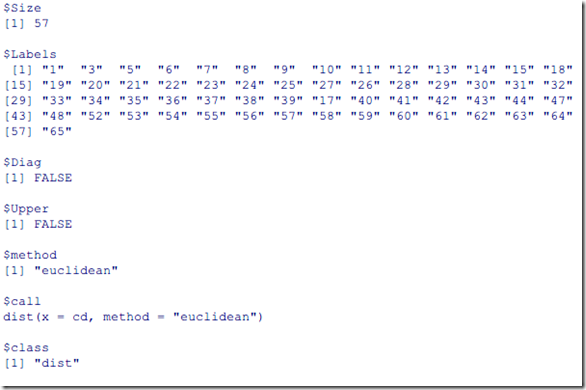• single: 两个聚类之间最近的点作为聚类的距离
• complete：两个聚类之间最远的点作为聚类的距离
• average:将聚类中的所有向量之和的平局向量作为聚类中心点，中心点最近的聚合成一类。

averge聚类的效果介于single和complte之间，如下面命令，

 1 2 cave = hclust(cds, method="average") plot(cave,hang=-1)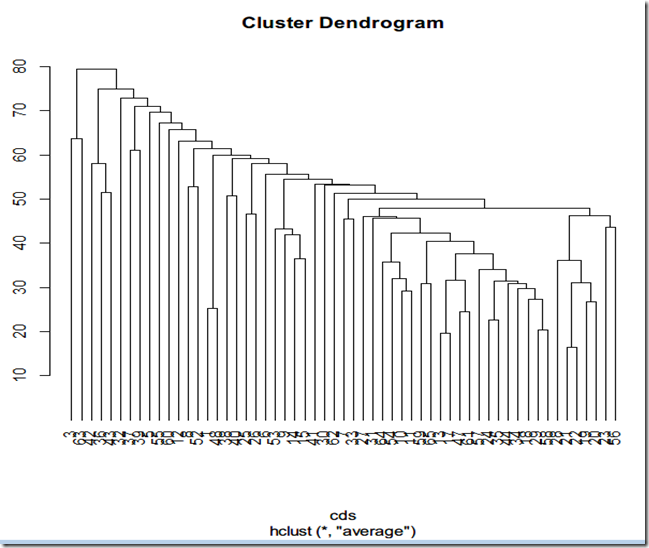1 2 cop = cophenetic(cave) cor(cop, cds)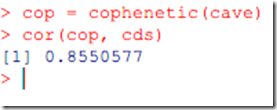1 2 3 4 5 6 7 8 cosine = function(a,b) {       len = (sqrt(a %*% a)*sqrt(b %*% b));       if (len == 0) {            0;       } else {            (a %*% b)/len;       }  }

（是不是写法有点像JS）

 1 library(proxy)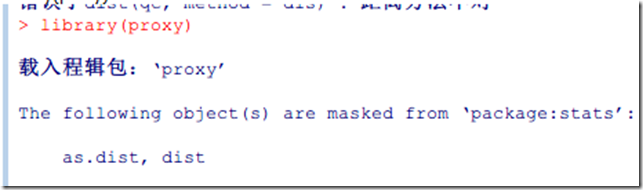1 cds = dist(t,method="cosine")

 1 2 3 4 5 6 7 8 9 10 11 12 13 disFunc = c("euclidean", "maximum", "manhattan" , "canberra", "binary", "minkowski");  #disFunc = c("euclidean", "cosine");  cluFunc = c("complete",     "single", "average", "ward", "mcquitty", "median", "centroid");  qc = read.table("d:/cluster_data.txt", header = TRUE);  for (dis in disFunc) {       for (clu in cluFunc) {            qcDis = dist(qc, method=dis);            c = hclust(qcDis, method=clu);            cop = cophenetic(c);            r = cor(cop,qcDis);            print(paste(dis, clu, r, sep=" "));       }  }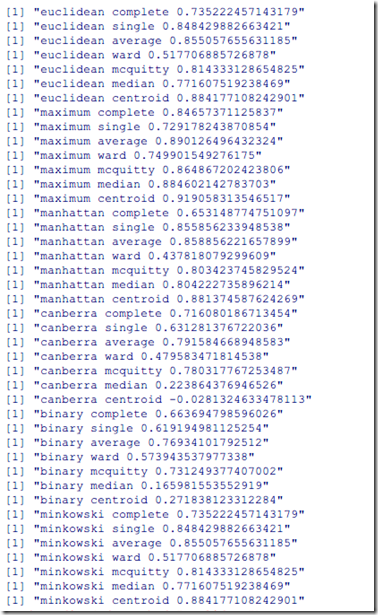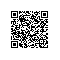使用钉钉扫一扫加入圈子
+ 订阅